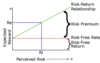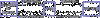Capital Budgeting: Intro and Projection Risk Flashcards Preview

BEC > Capital Budgeting: Intro and Projection Risk > Flashcards

Flashcards in Capital Budgeting: Intro and Projection Risk Deck (10):
1

2

3

Risk Reward Relationship:

Risk Reward Relationship: The greater the perceived risk, the greater the expected reward. Thus, the relationship between risk and reward is positive and can be shown graphically as:4

Define "risk-free rate of return".

5

Risk Premium:The rate of return expected above the risk-free rate based on the perceived level of risk inherent in an investment/undertaking. The Award.6

Describe the relationship between a firm's capital projects and the firm's capital that funds those projects.

The rate of return earned on a firm's capital project must be equal or greater than the rate of return required to attract and maintain investors' capital.7

8

9

Question:As the perceived risk of an undertaking increases, what would be the expected effect on the risk-free rate of return and the risk premium rate of return? Risk-Free Rate   Risk Premium     Increase  Increase    No change  Increase    No change  No change    Decrease  Decrease

Risk-Free Rate   Risk Premium  No change  Increase   The risk-free rate is the reward expected for deferring current consumption and does not change as the perceived risk of an undertaking increases (or decreases). Therefore, no change would be expected in the risk-free rate. An increase in the risk premium would be expected as a result of an increase in perceived risk; it is the "reward" for risk. Thus, the greater the risk, the greater the expected reward (or risk premium).10